# MCAT General Chemistry Practice Test 6: Equilibrium

### Test Information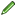15 questions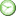minutes

Take more free MCAT general chemistry practice tests available from maintests.com.

1. A reaction is found to stop just before all reactants are converted to products. Which of the following could be true about this reaction?

• A. The reaction is irreversible, and the forward rate is greater than the reverse rate.
• B. The reaction is irreversible, and the reverse rate is too large for products to form.
• C. The reaction is reversible, and the forward rate is equal to the reverse rate.
• D. The reaction is reversible, and the reverse rate is greater than the forward rate.

2. What is the equilibrium expression for the reaction Cu2SO4 (s) ? 2 Cu+ (aq) + SO42- (aq)?

• A.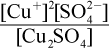• B.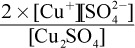• C.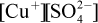• D.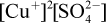3. Carbonated beverages are produced by dissolving carbon dioxide in water to produce carbonic acid:

CO2 (g) + H2O (l) ? H2CO3 (aq)

When a bottle containing carbonated water is opened, the taste of the beverage gradually changes as the carbonation is lost. Which of the following statements best explains this phenomenon?

• A. The change in pressure and volume causes the reaction to shift to the left, thereby decreasing the amount of aqueous carbonic acid.
• B. The change in pressure and volume causes the reaction to shift to the right, thereby decreasing the amount of gaseous carbon dioxide.
• C. Carbonic acid reacts with environmental oxygen and nitrogen.
• D. Carbon dioxide reacts with environmental oxygen and nitrogen.

4. What is the proper equilibrium expression for the reaction below?

2 NO2 (g) + 4 H2 (g) ? N2 (g) + 4 H2O (g)

• A.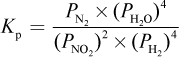• B.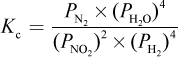• C.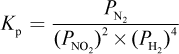• D.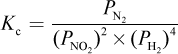5. If Kc ? 1:

• A. the equilibrium mixture will favor products over reactants.
• B. the equilibrium mixture will favor reactants over products.
• C. the equilibrium concentrations of reactants and products are equal.
• D. the reaction is essentially irreversible.

6. Acetic acid dissociates in solution according to the following equation:

CH3COOH ? CH3COO + H+

If sodium acetate is added to a solution of acetic acid in excess water, which of the following effects would be observed in the solution?

• A. Decreased pH
• B. Increased pH
• C. Decreased pKeq (pKa)
• D. Increased pKeq (pKa)

7. Given the reaction below:

FeI (aq) + I2 (g) → FeI3 (aq)

Which of the following would increase the formation of product?

• A. Decreasing the volume of the container
• B. Decreasing the pressure of the container
• C. Increasing the volume of the container
• D. Decreasing the volume of the container while maintaining a constant pressure

8. If the reaction FeI (aq) + I2 (g) → FeI3 (aq) were exothermic, what effect would decreasing the temperature have on the equilibrium?

• A. The forward reaction rate and the reverse reaction rate both increase.
• B. The forward reaction rate decreases while the reverse reaction rate increases.
• C. The forward reaction rate increases while the reverse reaction rate decreases.
• D. The forward reaction rate and the reverse reaction rate both decrease.

9. Which of the following actions does NOT affect the equilibrium position of a reaction?

• A. Adding or subtracting heat.
• B. Adding or removing a catalyst.
• C. Increasing or decreasing concentrations of reactants.
• D. Increasing or decreasing volumes of reactants.

10. In a sealed 1 L container, 1 mole of nitrogen gas reacts with 3 moles of hydrogen gas to form 0.05 moles of NH3 at equilibrium. Which of the following is closest to the Kc of the reaction?

• A. 0.0001
• B. 0.001
• C. 0.01
• D. 0.1

11. Increasing temperature can alter the Keq of a reaction. Why might increasing temperature indefinitely be unfavorable for changing reaction conditions?

• A. The equilibrium constant has a definite limit that cannot be surpassed.
• B. The products or reactants can decompose at high temperatures.
• C. Increasing temperature would decrease pressure, which may or may not alter reaction conditions.
• D. If a reaction is irreversible, its Keq will resist changes in temperature.

12. Which of the following is true of equilibrium reactions?

I. An increase in k1 results in a decrease in k–1.

II. As the concentration of products increases, the concentrations of reactants decreases.

III. The equilibrium constant is altered by changes in temperature.

• A. I only
• B. II and III only
• C. I and III only
• D. I, II, and III

13. Compound A has a Ka (equilibrium constant of acid dissociation) of approximately 10–4. Which of the following compounds is most likely to react with a solution of compound A?

• A. HNO3
• B. NO2
• C. NH3
• D. N2O5

14. Consider the following two reactions:

3 A + 2 B ? 3 C + 4 D (Reaction 1)

4 D + 3 C ? 3 A + 2 B (Reaction 2)

If Keq for reaction 1 is equal to 0.1, what is Keq for reaction 2?

• A. 0.1
• B. 1
• C. 10
• D. 100

15. Which of the following statements best describes the effect of lowering the temperature of the following reaction?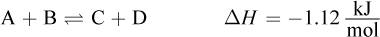• A. [C] and [D] would increase.
• B. [A] and [B] would increase.
• C. ΔH would increase.
• D. ΔH would decrease.

﻿